``````library(ggplot2) # for general plotting
library(car)     # for ANOVA (Type II used, better than Type I when there is an unbalanced design)``````

## Information of data source

Enzyme inhibitors were used to treat mites, fecundity and enzyme activity following enzyme inhibition were assessed.

Inhibitors:

PBO - CYP (cytochrome P450) inhibitor

DEM - GST inhibitor

DEF - esterase inhibitor

## Read in the data and view structure to identify any issues in data formatting

``````DEM.data <- read.csv("~/Lab Stuff/Adapted mites/Tomato/Inhibitor assay/Thesis - enzyme activity/DEM/TA DEM enzyme activity R data.csv", header = TRUE)

# Trial as a factor
DEM.data\$Trial <- factor(DEM.data\$Trial)

str(DEM.data)``````
``````## 'data.frame':    38 obs. of  4 variables:
##  \$ Trial      : Factor w/ 2 levels "1","2": 1 1 1 1 1 1 1 1 1 1 ...
##  \$ Mite.Strain: Factor w/ 2 levels "TU","TU-A": 1 1 1 1 1 1 1 1 1 2 ...
##  \$ Treatment  : Factor w/ 2 levels "Control","DEM": 1 1 1 1 1 2 2 2 2 1 ...
##  \$ Activity   : num  7.84 9.2 8.26 5.78 8.13 ...``````

## Formulate hypothesis

H0: There will be no difference in enzyme activity.

HA: Treatment with detoxification enzyme inhibitor will result in degreased enzyme activity.

## Conduct data exploration

### Outliers in the response variable (`Activity`) within explanatory variables (`Trial`, `Treatment`, `Mite.Strain`).

``ggplot(DEM.data, aes(x = Trial, y = Activity)) + geom_boxplot()+ theme_classic()``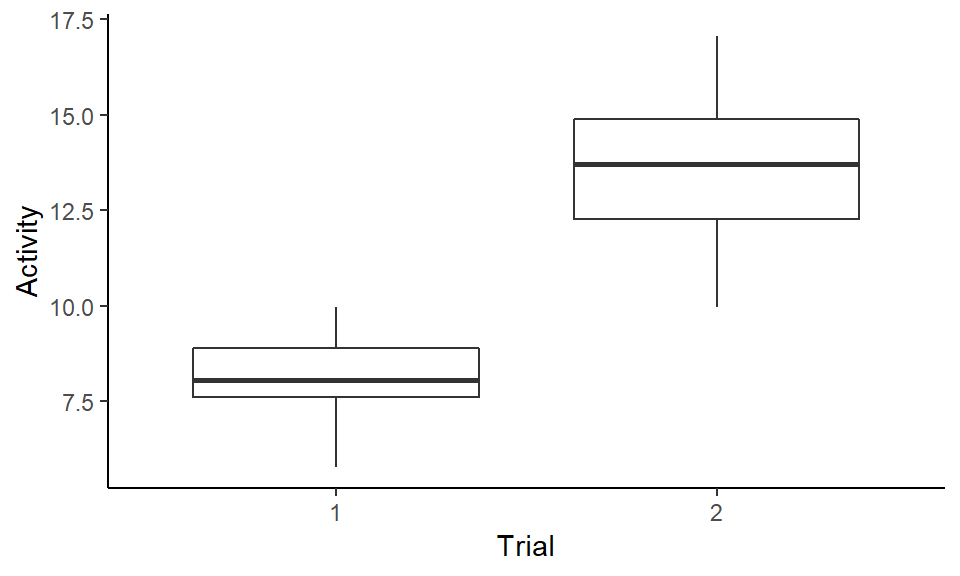``ggplot(DEM.data, aes(x = Treatment, y = Activity)) + geom_boxplot()+ theme_classic() ``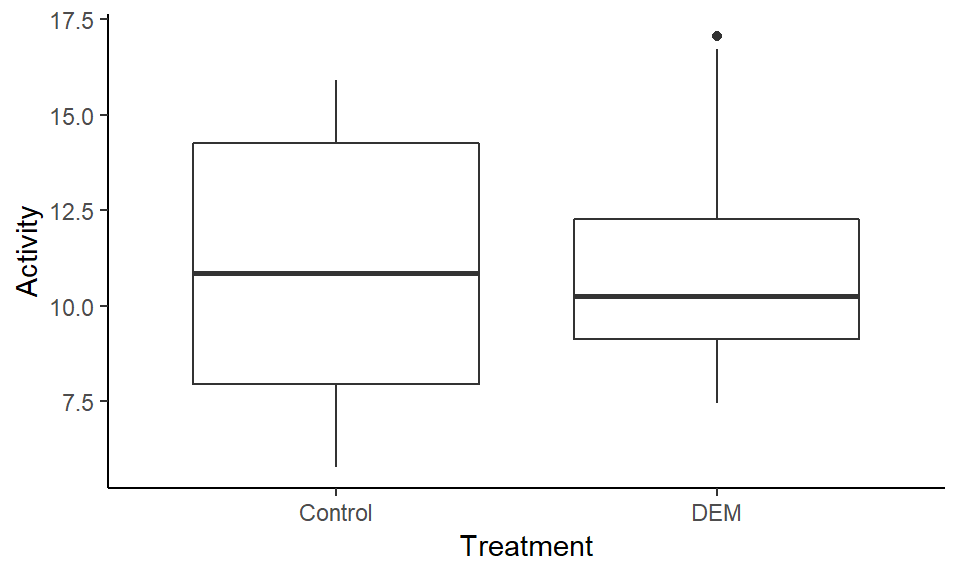``ggplot(DEM.data, aes(x = Mite.Strain, y = Activity)) + geom_boxplot() + theme_classic()``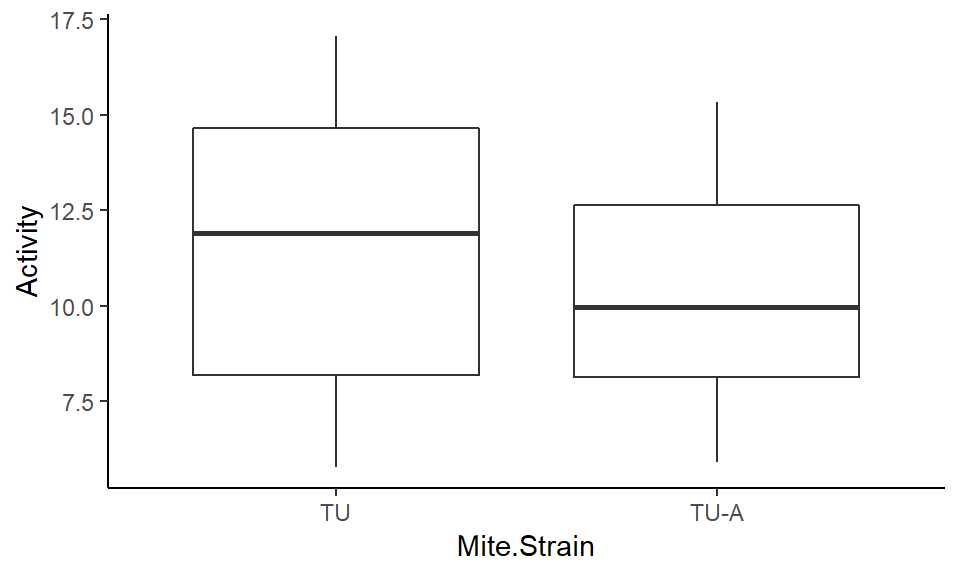Outlier left in, probably represents real variability.

### Collinearity of the explanatory variables

Does not apply, all explanatory variables are categorical/factorial.

### Spatial/temporal or other hierarchical aspects of sampling design

No, I am treating `Trial` as a main effect to check for reproducibility (not a random effect/blocking factor).

### Interactions (is the quality of the data good enough to include them?)

Interaction between`Trial` and `Mite.Strain` will be performed to test for reproducibility.

Interaction between`Trial` and `Treatment` will be performed to test for reproducibility.

Interaction between`Mite.Strain` and `Treatment` will be performed to check if the mite strains responded differently to treatment.

No

### Are categorical covariates balanced?

``summary(DEM.data)``
``````##  Trial  Mite.Strain   Treatment     Activity
##  1:18   TU  :19     Control:20   Min.   : 5.784
##  2:20   TU-A:19     DEM    :18   1st Qu.: 8.162
##                                  Median :10.245
##                                  Mean   :11.011
##                                  3rd Qu.:13.713
##                                  Max.   :17.062``````

Not in all cases, but they are close.

## Apply model

``````# fit linear model and display model fit information and ANOVA table
# full model including 3 way interaction term - to verify it is not significant, if it is, interpretation of hypothesis testing will be problematic
m.0 <- lm(Activity ~ Mite.Strain + Treatment + Trial + Mite.Strain:Treatment + Treatment:Trial + Mite.Strain:Trial + Mite.Strain:Treatment:Trial, data = DEM.data)
summary(m.0)``````
``````##
## Call:
## lm(formula = Activity ~ Mite.Strain + Treatment + Trial + Mite.Strain:Treatment +
##     Treatment:Trial + Mite.Strain:Trial + Mite.Strain:Treatment:Trial,
##     data = DEM.data)
##
## Residuals:
##     Min      1Q  Median      3Q     Max
## -2.3596 -0.9002  0.1777  0.7345  2.7994
##
## Coefficients:
##                                     Estimate Std. Error t value Pr(>|t|)
## (Intercept)                           7.8414     0.6066  12.927 8.51e-14
## Mite.StrainTU-A                      -0.3206     0.8579  -0.374    0.711
## TreatmentDEM                          0.3581     0.9099   0.394    0.697
## Trial2                                7.0590     0.8579   8.228 3.48e-09
## Mite.StrainTU-A:TreatmentDEM          1.1934     1.2868   0.927    0.361
## TreatmentDEM:Trial2                  -0.9959     1.2506  -0.796    0.432
## Mite.StrainTU-A:Trial2               -0.8404     1.2132  -0.693    0.494
## Mite.StrainTU-A:TreatmentDEM:Trial2  -2.6908     1.7686  -1.521    0.139
##
## (Intercept)                         ***
## Mite.StrainTU-A
## TreatmentDEM
## Trial2                              ***
## Mite.StrainTU-A:TreatmentDEM
## TreatmentDEM:Trial2
## Mite.StrainTU-A:Trial2
## Mite.StrainTU-A:TreatmentDEM:Trial2
## ---
## Signif. codes:  0 '***' 0.001 '**' 0.01 '*' 0.05 '.' 0.1 ' ' 1
##
## Residual standard error: 1.356 on 30 degrees of freedom
## Multiple R-squared:  0.8549, Adjusted R-squared:  0.8211
## F-statistic: 25.26 on 7 and 30 DF,  p-value: 6.35e-11``````
``Anova(m.0)``
``````## Anova Table (Type II tests)
##
## Response: Activity
##                              Sum Sq Df  F value    Pr(>F)
## Mite.Strain                   7.793  1   4.2359   0.04836 *
## Treatment                     0.766  1   0.4161   0.52377
## Trial                       289.554  1 157.3774 1.825e-13 ***
## Mite.Strain:Treatment         0.126  1   0.0686   0.79521
## Treatment:Trial              12.898  1   7.0102   0.01279 *
## Mite.Strain:Trial            10.478  1   5.6952   0.02352 *
## Mite.Strain:Treatment:Trial   4.259  1   2.3148   0.13862
## Residuals                    55.196 30
## ---
## Signif. codes:  0 '***' 0.001 '**' 0.01 '*' 0.05 '.' 0.1 ' ' 1``````
``````# linear model without non-significant 3-way interaction (want to reduce comparisons made in Tukey-Kramer post-hoc test)
m <- lm(Activity ~ Mite.Strain + Treatment + Trial + Mite.Strain:Treatment + Treatment:Trial + Mite.Strain:Trial, data = DEM.data)
summary(m)``````
``````##
## Call:
## lm(formula = Activity ~ Mite.Strain + Treatment + Trial + Mite.Strain:Treatment +
##     Treatment:Trial + Mite.Strain:Trial, data = DEM.data)
##
## Residuals:
##      Min       1Q   Median       3Q      Max
## -2.04304 -0.87577  0.09814  0.67314  3.11596
##
## Coefficients:
##                              Estimate Std. Error t value Pr(>|t|)
## (Intercept)                    7.5248     0.5818  12.934 4.95e-14 ***
## Mite.StrainTU-A                0.3125     0.7659   0.408   0.6861
## TreatmentDEM                   1.0704     0.7966   1.344   0.1888
## Trial2                         7.6921     0.7659  10.043 2.89e-11 ***
## Mite.StrainTU-A:TreatmentDEM  -0.2312     0.9013  -0.256   0.7993
## TreatmentDEM:Trial2           -2.3413     0.9028  -2.593   0.0144 *
## Mite.StrainTU-A:Trial2        -2.1066     0.9013  -2.337   0.0261 *
## ---
## Signif. codes:  0 '***' 0.001 '**' 0.01 '*' 0.05 '.' 0.1 ' ' 1
##
## Residual standard error: 1.385 on 31 degrees of freedom
## Multiple R-squared:  0.8437, Adjusted R-squared:  0.8135
## F-statistic:  27.9 on 6 and 31 DF,  p-value: 3.362e-11``````
``Anova(m)``
``````## Anova Table (Type II tests)
##
## Response: Activity
##                        Sum Sq Df  F value    Pr(>F)
## Mite.Strain             7.793  1   4.0635   0.05256 .
## Treatment               0.766  1   0.3992   0.53213
## Trial                 289.554  1 150.9742 1.891e-13 ***
## Mite.Strain:Treatment   0.126  1   0.0658   0.79927
## Treatment:Trial        12.898  1   6.7250   0.01438 *
## Mite.Strain:Trial      10.478  1   5.4635   0.02605 *
## Residuals              59.455 31
## ---
## Signif. codes:  0 '***' 0.001 '**' 0.01 '*' 0.05 '.' 0.1 ' ' 1``````
``````# Calculate effect size and display
result.anova<-Anova(m)
ss<-result.anova\$"Sum Sq"    ##ss = sum of squares
pes<-ss/(ss+ss[length(ss)])  ##pes = partial e squared
pes[length(pes)]<-""
result.anova\$"Part E Sq"<-pes
result.anova``````
``````## Anova Table (Type II tests)
##
## Response: Activity
##                        Sum Sq Df  F value  Pr(>F) Part E Sq
## Mite.Strain             7.793  1   4.0635 0.05256   0.11589
## Treatment               0.766  1   0.3992 0.53213   0.01271
## Trial                 289.554  1 150.9742 0.00000   0.82965
## Mite.Strain:Treatment   0.126  1   0.0658 0.79927   0.00212
## Treatment:Trial        12.898  1   6.7250 0.01438   0.17826
## Mite.Strain:Trial      10.478  1   5.4635 0.02605   0.14983
## Residuals              59.455 31``````
``````# plot interactions
interaction.plot(DEM.data\$Mite.Strain, DEM.data\$Treatment, DEM.data\$Activity, type="l", leg.bty="o", leg.bg="grey95", lwd=2, ylab="Activity", xlab="Mite.Strain", main="Mite.Strain:Treatment")``````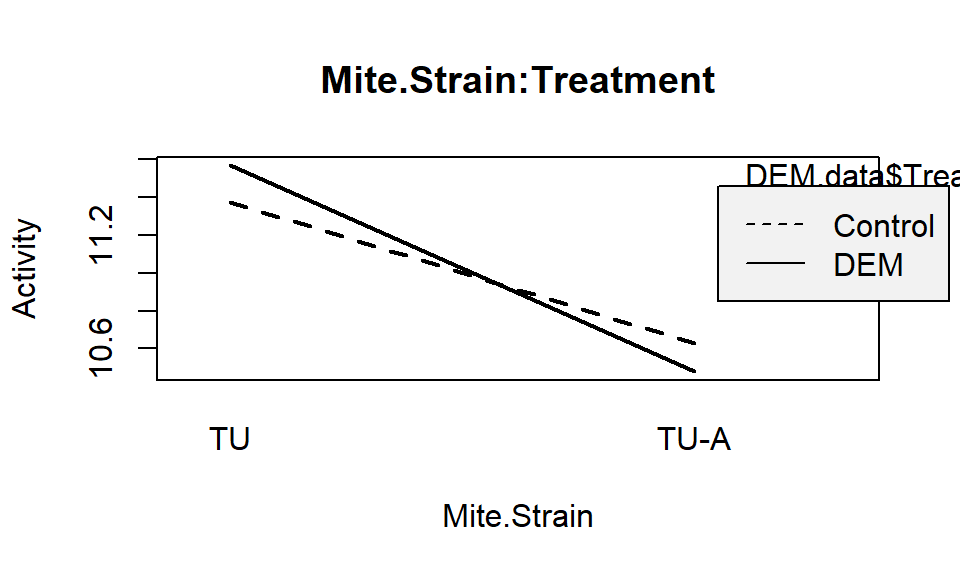``interaction.plot(DEM.data\$Treatment, DEM.data\$Trial, DEM.data\$Activity, type="l", leg.bty="o", leg.bg="grey95", lwd=2, ylab="Activity", xlab="Treatment", main="Treatment:Trial")``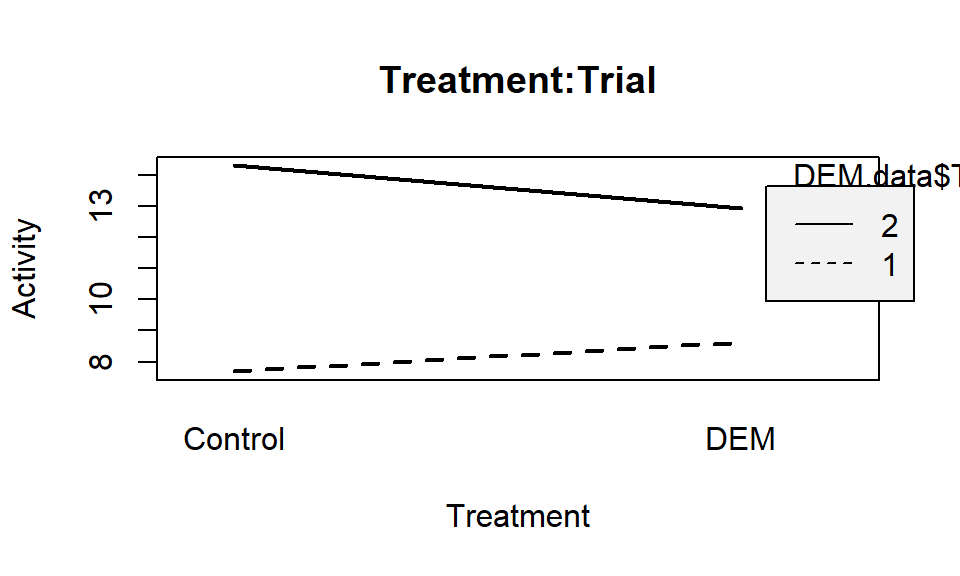``interaction.plot(DEM.data\$Mite.Strain, DEM.data\$Trial, DEM.data\$Activity, type="l", leg.bty="o", leg.bg="grey95", lwd=2, ylab="Activity", xlab="Mite.Strain", main="Mite.Strain:Trial")``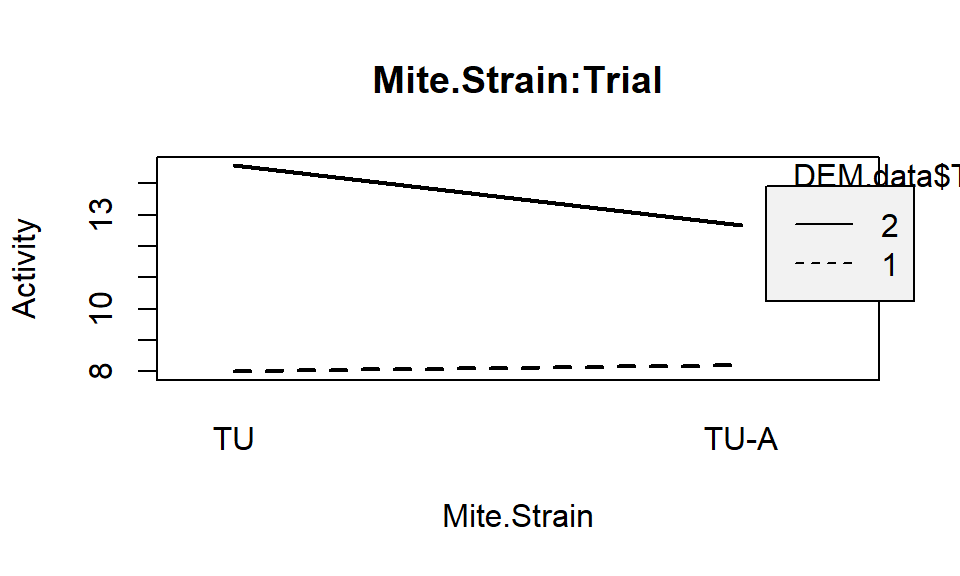## Validate model

``````DEM.data\$m.fit <- fitted(m) # fitted values
DEM.data\$m.res <- rstandard(m) # Pearson residuals``````

### Residual distribution / Overdispersion

We assumed normal residuals. This is the least important regression assumption but its can be tested with a qq plot.

``````ggplot(DEM.data, aes(sample = m.res)) + geom_qq() +
geom_abline(intercept = 0, slope = 1) + theme_classic()``````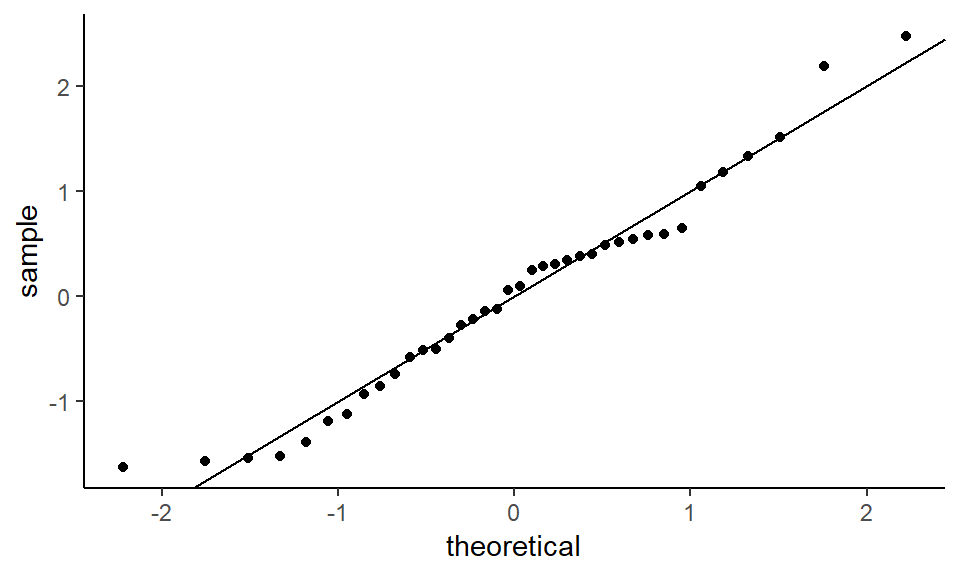Nice.

### Residuals vs fitted values

Testing for:

Linearity - there should be no curvilinear pattern in the residuals.

Equal variance - the vertical spread of the residuals should be constant across all fitted values.

``````ggplot(DEM.data, aes(x = m.fit, y = m.res)) +
geom_point() + geom_hline(yintercept = 0) + geom_smooth()``````
``## `geom_smooth()` using method = 'loess' and formula 'y ~ x'``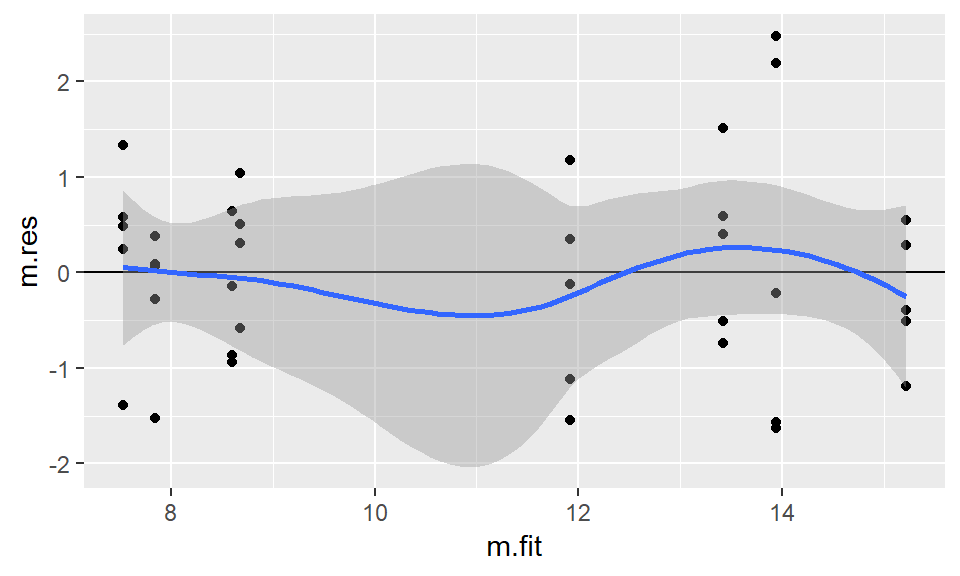Little curvature, confidence intervals always include 0. Spread of variables looks pretty good.

### Residuals vs explanatory variables

Should be centered around 0, if not then model requires another explanatory variable(s), to account for observed variation.

``````ggplot(DEM.data, aes(x = Trial, y = m.res)) +
geom_boxplot() + theme_classic() + geom_hline(yintercept = 0) ``````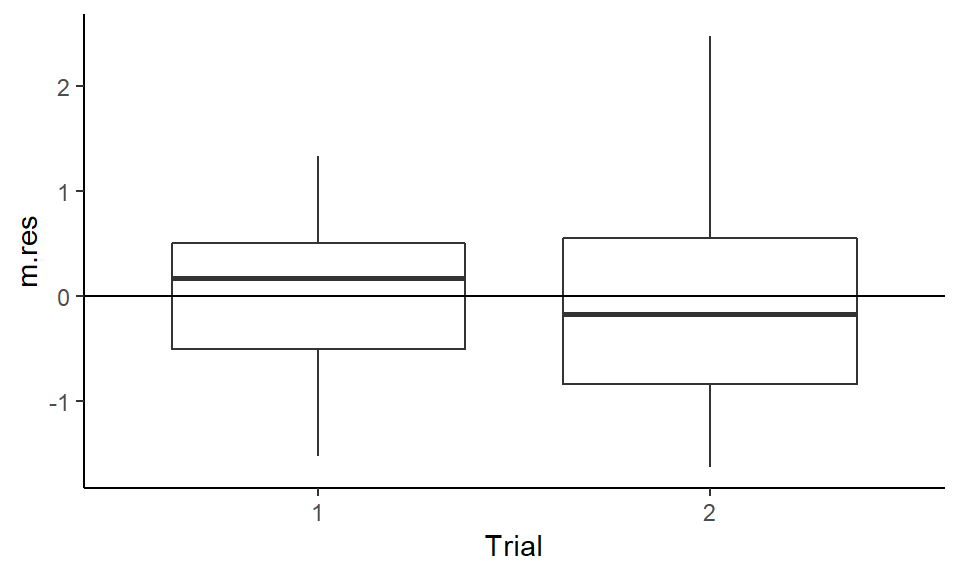``````ggplot(DEM.data, aes(x = Treatment, y = m.res)) +
geom_boxplot() + theme_classic() + geom_hline(yintercept = 0) ``````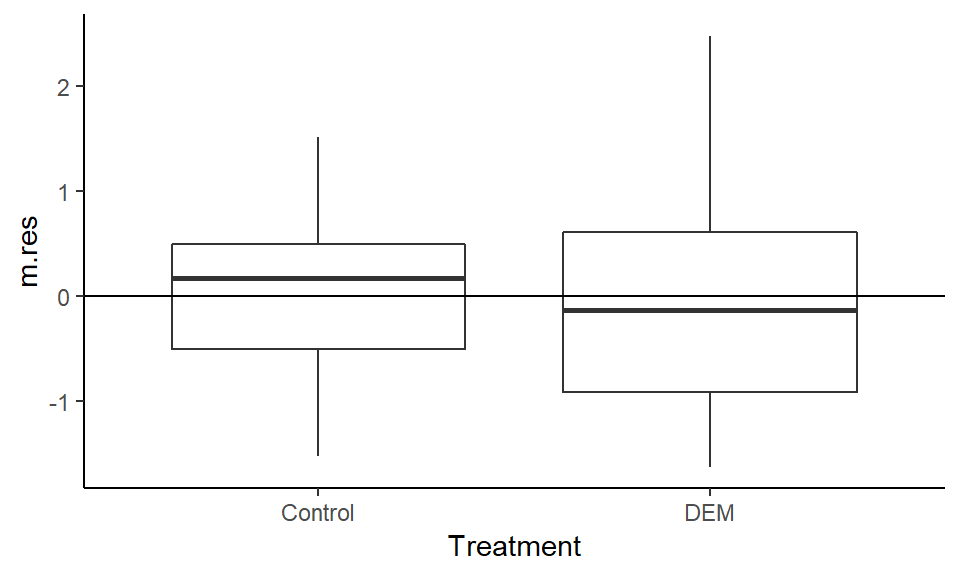``````ggplot(DEM.data, aes(x = Mite.Strain, y = m.res)) +
geom_boxplot() + theme_classic() + geom_hline(yintercept = 0) ``````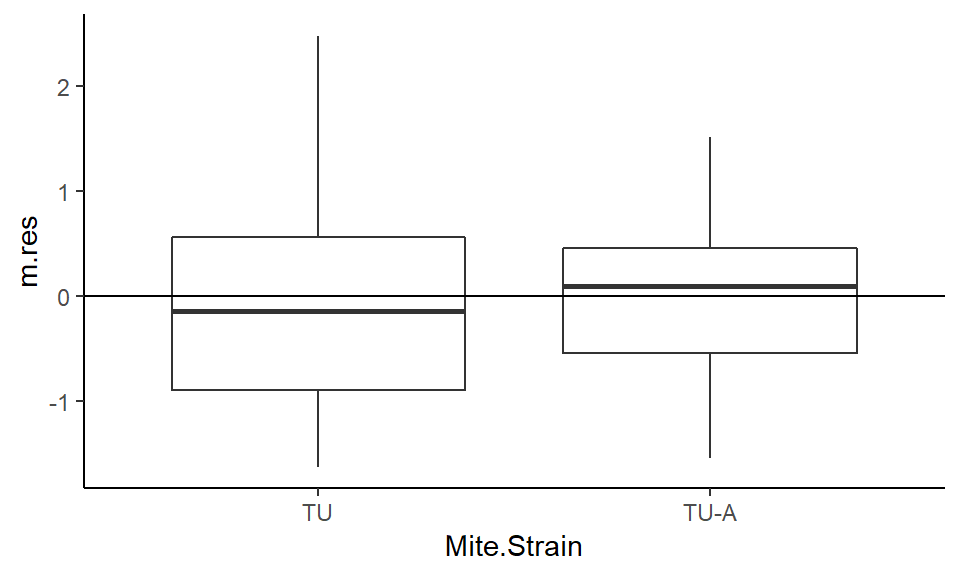``````ggplot(DEM.data, aes(x = Mite.Strain:Trial, y = m.res)) +
geom_boxplot() + theme_classic() + geom_hline(yintercept = 0) ``````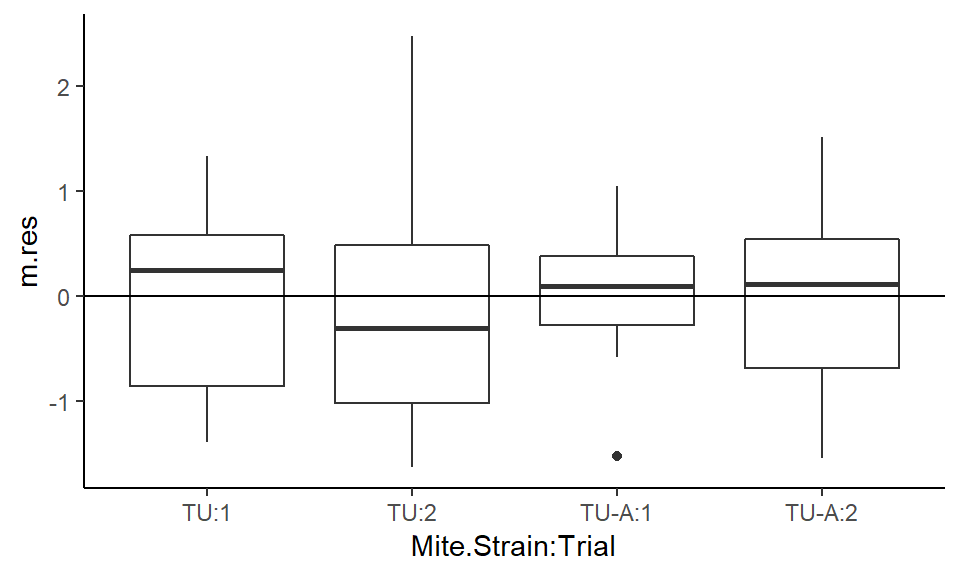``````ggplot(DEM.data, aes(x = Treatment:Trial, y = m.res)) +
geom_boxplot() + theme_classic() + geom_hline(yintercept = 0)``````